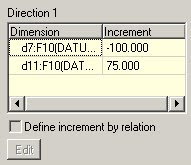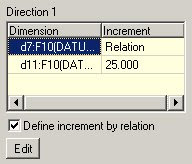### Answer to the ProE Puzzle 001

Here is my answer for the proe puzzle_001.
I must admit that, I could not fully solve my Qn. I will come to that later.

Here is the sequence that I followed.

1. I created two points, one on X-Axis and the other on Y-Axis. (I used Offset_Co-ordinate_System Option. Insert -> Model Datum -> Point).

2. Created a pattern of these points. I select the X-Axis distance of first point and Y-Axis distance of the second point in the Direction1 of the pattern. This is the KEY point of the puzzle. This way both dimension would be incremented to get each item of the pattern. The result would completely be different if the second dimension is part of Direction 2. Anyway try it out yourself if you are still not clear. This can be very useful as any dimension can be included in this way.3. Create a curve (using Thru Points option) between the first two points that you created in Step 1.

4. Pattern this curve with the option, Ref Pattern.

5. Create a point on the curve you created in step 3 at the mid point.

6. Pattern this point with the option, Ref Pattern.

7. Now you have a set of points that you can use for creating your final curve.Here you can change the no of pattern elements, increment in X-Axis and increment in Y-Axis in the pattern of Step 2.

Here is a problem. If I increase or decrease the no of pattern elements (of step 2), I need to redefine the curve of step 7. I don't know how that can also be made dynamically updatable. Hope some one has a suggestion.

More options:

Now, I use this to show you another option of ProE pattern. That's is to use Relations in pattern. The modification is in the Step 2. Instead of having a constant increment, I can have a variable increment, controlled through relation.In the picture you can see that X1 is not equal to X2. what I did was this. I control in increment in X-Axis by relation. Select the dimension you want to control through relation and click Edit (refer the picture).the relation I used is this.
memb_i = -2 * (idx1*idx1)
where memb_i is the increment and idx1 is the instance no.

for eg...
the distance between the 1st point and second point will be -2 * (1 * 1) = -2
the distance between the 5th point and 6th point will be -2 * (5 * 5) = -50
and so on..

Other option are...
you can use memb_v instead of memb_i. memb_v will be the resultant dimension instead of the increment.
If you have Direction 2 also, then you have the option of using idx2 also in this relation.

### TreeView Structure in Excel VBA

TreeView is extreamly useful in specific cases but it can be bit tricky sometimes to implement.  Last Few days I was working on a TreeView Structure and thought I will share the knowledge I gained...  This post takes you through the basic operations to create and operate a TreeView. It will be like the Folder tree window of the windows explorer.  Moreover, you can have it dynamically updated based on the data in excel.... Here we go..

### Excel Function :TABLE

Its been a while since I have done some posting. This option in excel is something which I thought would be very useful for many. Its the TABLE Function in MS Excel. This is especially useful in the following scenarios.

### Proe Function / Relation to round the decimal places

Its strange that ProE does not have a ROUND function. At least I counldnt find it. It has two funtions. Ceil will always round it up. Floor will always round it down. I have used both to get the ROUND functionality. here is the relation...